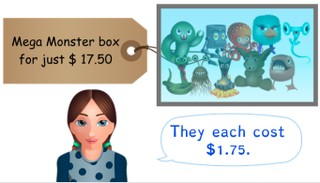Dividing by 10, 100 and 1,000 with decimal numbers

# Dividing by 10, 100 and 1,000 with decimal numbers

Students learn to divide by 10, 100 and 1,000 with decimal numbers.8,000 schools use Gynzy92,000 teachers use Gynzy1,600,000 students use Gynzy

## General

The students learn to divide by 10, 100 and 1,000 with decimal numbers.

## Standards

CCSS.Math.Content.5.NBT.A.2

## Learning objective

Students will be able to divide numbers by 10, 100 or 1,000, for which the outcome is a decimal number.

## Introduction

The students write the decimal numbers in the correct places on the table.

## Instruction

Show what happens with the decimal point when you divide by 10. The decimal point moves one place to the left. So 88 ÷ 10 becomes 8.8. If there is no decimal point in the number yet, for example with 4 ÷ 10, then you put a zero and a decimal point in front of the number. If you are dividing by 100 with decimal numbers, then the decimal point moves two places to the left. In this way, 567 ÷ 100 = 5.67. If there is not yet a decimal point in the problem, for example with 23 ÷ 100, then a zero and a decimal point come before the number, and with each extra step another 0 comes after the decimal point. If you are dividing by 1,000, then the decimal point moves three places to the left. In this way, 5,900 ÷ 1,000 = 5.9. Practice the three problems together with the students and have them solve the following three problems on their own. Discuss the steps of a story problem, and have the students solve the next two story problems on their own.

Check whether the students can divide decimal numbers by 10, 100 and 1,000 by asking the following question:
- What happens to the decimal point if you divide by 100?

## Quiz

The students test their understanding of dividing by 10, 100 and 1,000 with decimal numbers through ten exercises. In each exercise the students must either divide by 10, 100 or 1,000, and their answer will be a decimal number. Some of the exercises are story problems.

## Closing

Discuss once again the importance of being able to divide by 10, 100 and 1,000 with decimal numbers. As a closing activity you have the students drag the monsters to the correct problems. The first monster represents dividing by 10. The second monster stands for dividing by 100, and the third monster for dividing by 1,000.

## Teaching tips

Have students that have difficulty with dividing by 10, 100 and 1,000 with decimal numbers first practice with structuring decimal numbers. Next you practice dividing by 10 and show what happens with the decimal point.

### The online teaching platform for interactive whiteboards and displays in schools

• Save time building lessons

• Manage the classroom more efficiently

• Increase student engagement